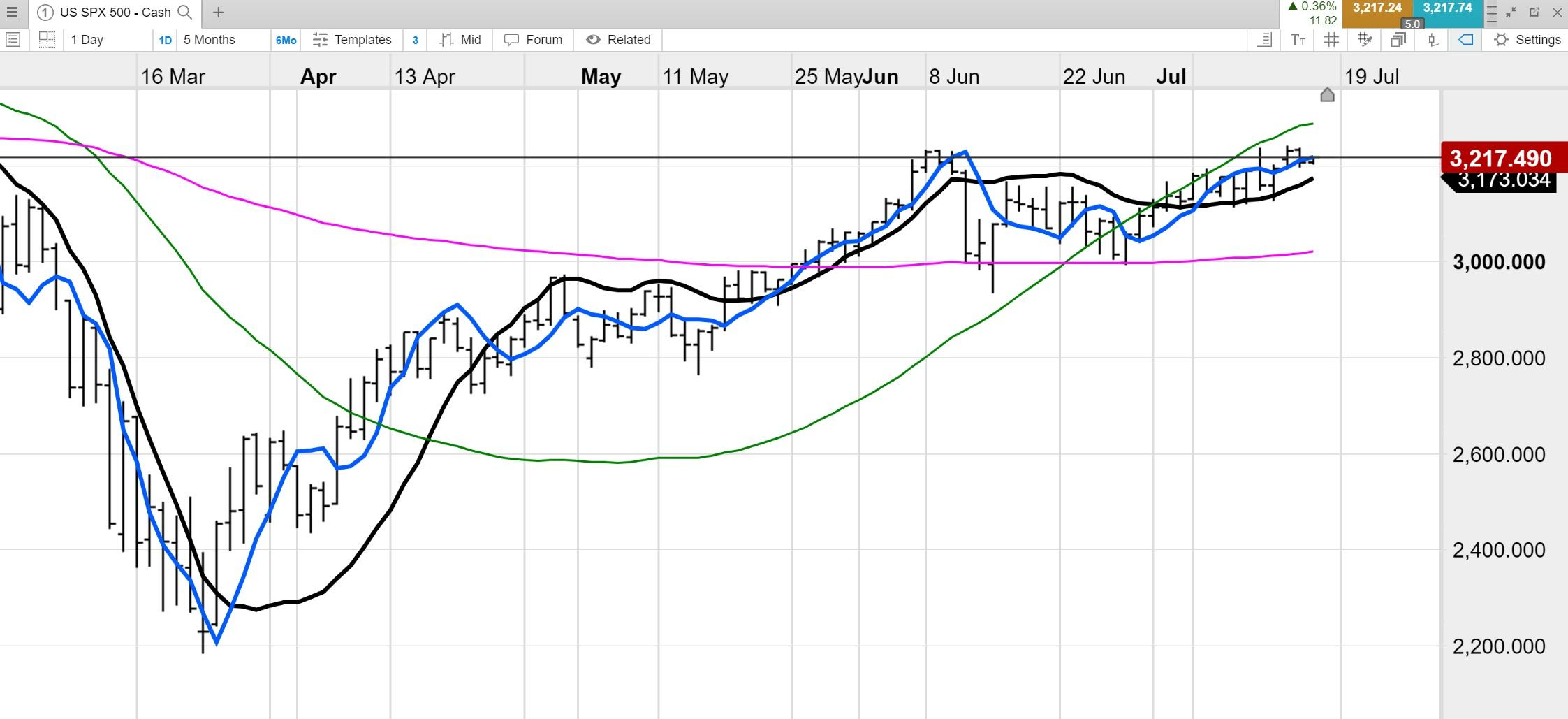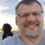Select Page

# Moving Linear Regression

Optuma Forums Optuma General Discussion Moving Linear Regression

Tagged:

Viewing 5 posts - 1 through 5 (of 5 total)
• Author
Posts
• #59025

Does Optuma have the Moving Linear Regression line?

if not, is there something similar?

Thanks

#59029

Hi Raul,

We have the Trailing Linear Regression tool which draws the regression line over the last X bars on a chart:

Trailing Linear Regression

We also have the Linear Regression Slope tool that calculates the slope of the line, so when it’s above zero the slope if positive:

Linear Regression Slope

If these are not what you require please provide a screenshot of what you are looking for.

Also, don’t forget that you can search for our tools either in the Tools menu of the software, or our online KnowledgeBase.

#59035

Thanks for your quick response, Darren.
I have checked the “Trailing Linear Regression” and the “Linear Regression Slope” indicators but is not the same.

The Moving Linear Regression is a moving average substitute. Please see image below

Pink line 270 period, Green line 90 period, Black line 30 period, Blue line 10 period.

Is it possible to convert the Linear Regression tool into a moving average with a script?#59073

Hi Raul,

We also have the Linear Regression Intercept tool:

https://help.optuma.com/kb/faq.php?id=529

#63219

Not sure if you got this sorted Raul, but I managed to put this together to get a Moving Linear Regression

For a 30 period use this in a SHOWPLOT:

Change out the 30’s for 10, 90, 270 etc

###### 2 users thanked author for this post.Darren,Julius
Viewing 5 posts - 1 through 5 (of 5 total)
• You must be logged in to reply to this topic.